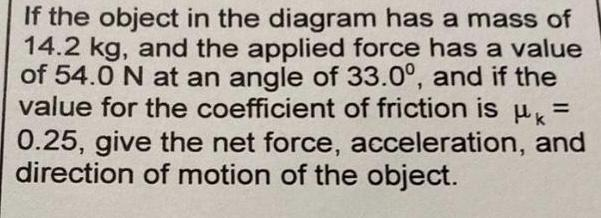Question:

# If the object in the diagram has a mass of 14.2 kg, and the

Last updated: 7/31/2022If the object in the diagram has a mass of 14.2 kg, and the applied force has a value of 54.0 N at an angle of 33.0°, and if the value for the coefficient of friction is μ = 0.25, give the net force, acceleration, and direction of motion of the object.